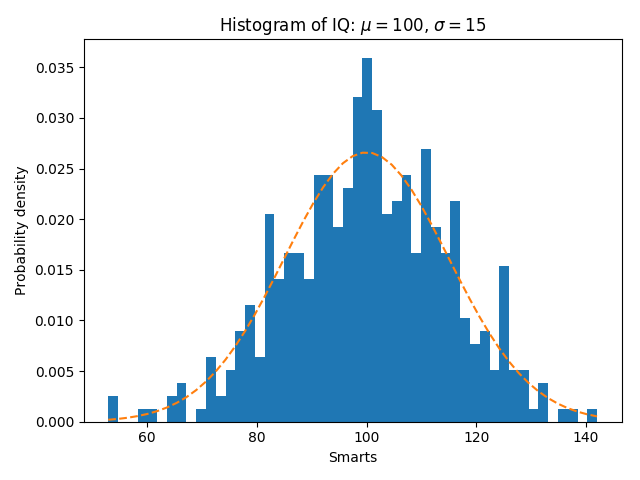Travis-CI:# Demo of the histogram (hist) function with a few features¶

In addition to the basic histogram, this demo shows a few optional features:

• Setting the number of data bins
• The normed flag, which normalizes bin heights so that the integral of the histogram is 1. The resulting histogram is an approximation of the probability density function.
• Setting the face color of the bars
• Setting the opacity (alpha value).

Selecting different bin counts and sizes can significantly affect the shape of a histogram. The Astropy docs have a great section on how to select these parameters: http://docs.astropy.org/en/stable/visualization/histogram.htmlimport numpy as np
import matplotlib.mlab as mlab
import matplotlib.pyplot as plt

np.random.seed(19680801)

# example data
mu = 100  # mean of distribution
sigma = 15  # standard deviation of distribution
x = mu + sigma * np.random.randn(437)

num_bins = 50

fig, ax = plt.subplots()

# the histogram of the data
n, bins, patches = ax.hist(x, num_bins, normed=1)

# add a 'best fit' line
y = mlab.normpdf(bins, mu, sigma)
ax.plot(bins, y, '--')
ax.set_xlabel('Smarts')
ax.set_ylabel('Probability density')
ax.set_title(r'Histogram of IQ: $\mu=100$, $\sigma=15$')

# Tweak spacing to prevent clipping of ylabel
fig.tight_layout()
plt.show()


Total running time of the script: ( 0 minutes 0.061 seconds)

Gallery generated by Sphinx-Gallery